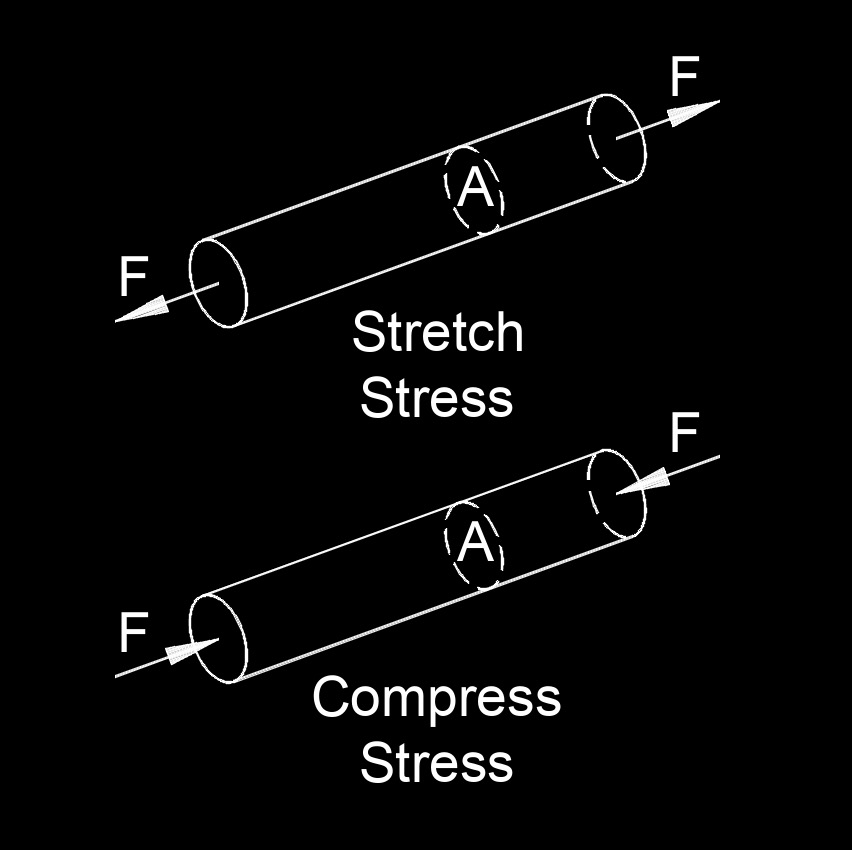# Elastic Modulus

Written by Jerry Ratzlaff on . Posted in Classical MechanicsElastic modulus (Young's modulus), abbreviated as $$\lambda$$ (Greek symbol lambda), also called modulus of elasticity, is the ratio of the stress applied to a body or substance to the resulting strain within the elastic limits.

## Elastic Modulus formula

 $$\large{ \lambda = \frac { \sigma } { \epsilon } }$$

### Where:

$$\large{ \lambda }$$  (Greek symbol lambda) = elastic modulus

$$\large{ \epsilon }$$  (Greek symbol epsilon) = strain

$$\large{ \sigma }$$  (Greek symbol sigma) = stress